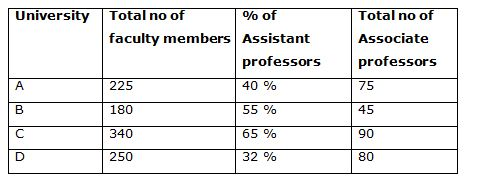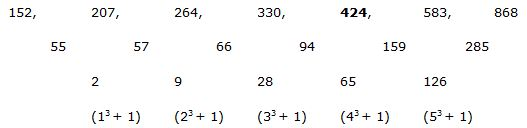# IBPS Clerk Prelims 2018 – Quantitative Aptitude Questions Day-32

Dear Readers, Bank Exam Race for the Year 2018 is already started, To enrich your preparation here we have providing new series of Practice Questions on Quantitative Aptitude – Section. Candidates those who are preparing for IBPS Clerk Prelims 2018 Exams can practice these questions daily and make your preparation effective.

[WpProQuiz 4341]

Directions (Q. 1 – 5): Find the wrong term in the following number series

1) 44, 22, 32.25, 82.5, 288.75, 1299.375

a) 82.5

b) 288.75

c) 32.25

d) 44

e) 22

2) 15, 16, 37, 117, 484, 2445

a) 37

b) 16

c) 2445

d) 484

e) 117

3) 2512, 632, 160, 44.5, 15.125

a) 632

b) 44.5

c) 15.125

d) 160

e) 2512

4) 504, 72, 990, 110, 1714, 156

a) 72

b) 1714

c) 990

d) 156

e) 110

5) 152, 207, 264, 330, 425, 583, 868

a) 207

b) 583

c) 425

d) 330

e) 868

Directions (Q. 6 – 10) Study the following information carefully and answer the given questions:Note: The faculty members include total no of assistant professors, total no of associate Professors and total no of professors.

6) Total number of assistant professors in University A is what percentage of total number of professors in University B?

a) 250 %

b) 178 %

c) 164 %

d) 200 %

e) None of these

7) Find the ratio between the total number of professors in university C to that of total number of associate professors in university B and D together?

a) 36: 115

b) 58: 131

c) 29: 125

d) 43: 189

e) None of these

8) Find the total number of assistant professors in all the universities together?

a) 370

b) 490

c) 520

d) 610

e) None of these

9) Total number of faculty members in university A and D together is approximately what percentage more/less than the total number of faculty members in university B and C together?

a) 33 %

b) 20 %

c) 9 %

d) 24 %

e) 42 %

10) In which university has highest number of professors?

a) University A

b) University B

c) University C

d) University D

e) None of these

Direction (1-5):

The correct series is,

44, 22, 33, 82.5, 288.75, 1299.375

The pattern is, *1/2, *3/2, *5/2, *7/2, *9/2,…

The wrong term is, 32.25

The correct series is,

15, 16, 36, 117, 484, 2445

The pattern is, *1 + 12, *2 + 22, *3 +32, *4 + 42, *5 + 52,…

The wrong term is, 37

The correct series is,

2512, 632, 162, 44.5, 15.125

The pattern is, ÷ 4 + 4

The wrong term is, 160

The correct series is,

504, 72, 990, 110, 1716, 156

The pattern is, 83 – 8, 92 – 9, 103 – 10, 112 – 11, 123 – 12, 132 – 13,..

The wrong term is, 1714

The correct series is,The difference of difference is, 13 + 1, 23 + 1, 33 +1, 43 +1, 53 +1,..

The wrong term is, 425

Direction (6-10):

Total number of assistant professors in University A

= > 225*(40/100) = 90

Total number of professors in University B

= > 180 – (55 % of 180 + 45)

= > 180 – (99 + 45) = 36

Required % = (90/36)*100 = 250 %

Total number of professors in university C

= > 340 – (65 % of 340 + 90)

= > 340 – (221 + 90) = 29

Total number of associate professors in university B and D together

= > 45 + 80 = 125

Required ratio = 29: 125

Total number of assistant professors in all universities

= > 225*(40/100) + 180*(55/100) + 340*(65/100) + 250*(32/100)

= > 90 + 99 + 221 + 80

= > 490

Total number of faculty members in university A and D

= > 225 + 250 = 475

Total number of faculty members in university B and C

= > 180 + 340 = 520

Less % = [(520-475)/520]*100 = 45*100/520 = 8.65 % = 9 %

Total number of professors in University A

= > 225 – (40 % of 225 + 75)

= > 225 – (90 + 75) = 60

Total number of professors in University B

= > 180 – (55 % of 180 + 45)

= > 180 – (99 + 45) = 36

Total number of professors in university C

= > 340 – (65 % of 340 + 90)

= > 340 – (221 + 90) = 29

Total number of professors in University D

= > 250 – (32 % of 250 + 80)

= > 250 – (80 + 80) = 90

University D has highest number of professors.

Kindly watch this video to know about Must Attempt Top 5 Arithmetic Problems for IBPS Clerk Prelims 2018

Daily Practice Test Schedule | Good Luck

 Topic Daily Publishing Time Daily News Papers & Editorials 8.00 AM Current Affairs Quiz 9.00 AM Current Affairs Quiz (Hindi) 9.30 AM IBPS Clerk Prelims – Reasoning 10.00 AM IBPS Clerk Prelims – Reasoning (Hindi) 10.30 AM IBPS Clerk Prelims – Quantitative Aptitude 11.00 AM IBPS Clerk Prelims – Quantitative Aptitude (Hindi) 11.30 AM Vocabulary (Based on The Hindu) 12.00 PM IBPS PO Prelims – English Language 1.00 PM SSC Practice Questions (Reasoning/Quantitative aptitude) 2.00 PM IBPS Clerk – GK Questions 3.00 PM SSC Practice Questions (English/General Knowledge) 4.00 PM Daily Current Affairs Updates 5.00 PM Canara Bank PO Mains – Reasoning 6.00 PM Canara Bank PO Mains – Quantitative Aptitude 7.00 PM Canara Bank PO Mains – English Language 8.00 PM